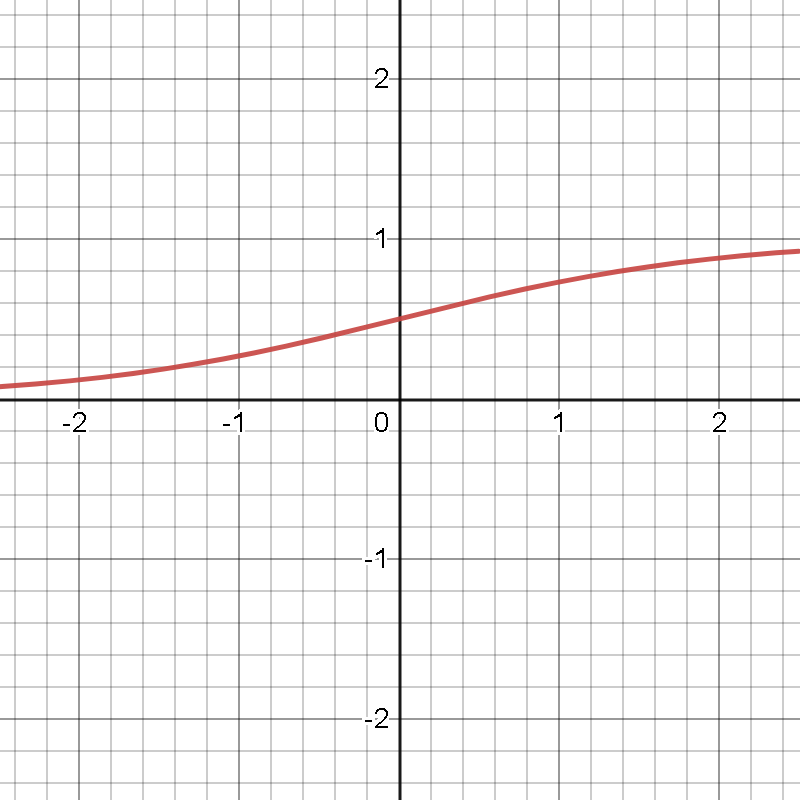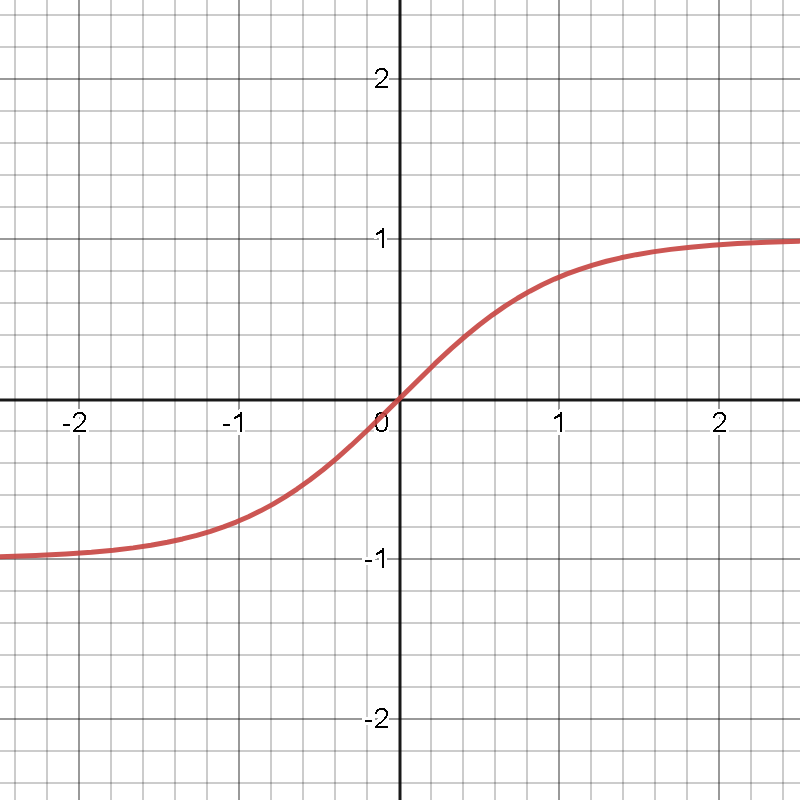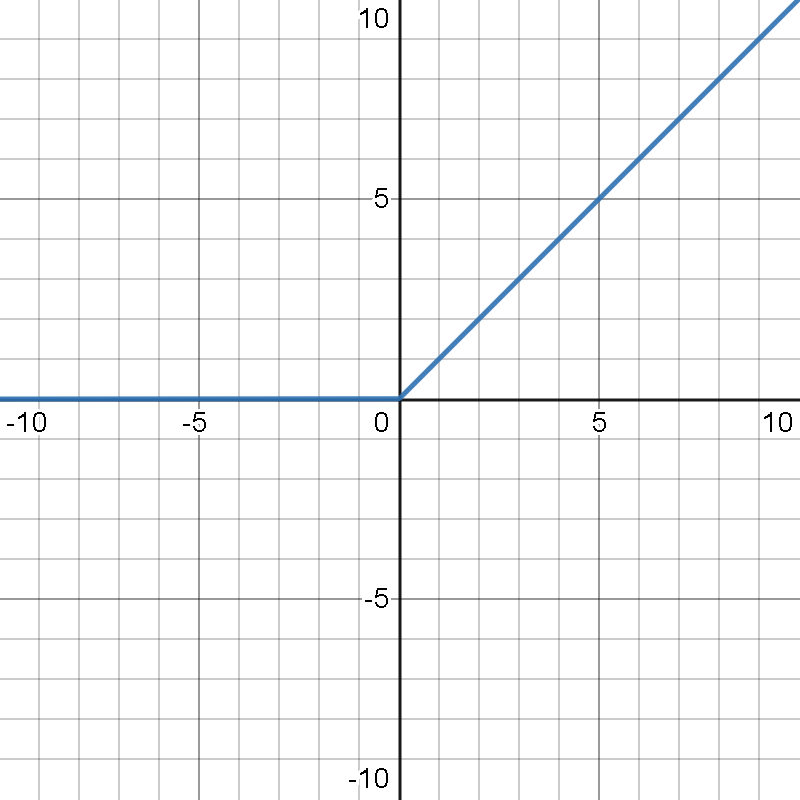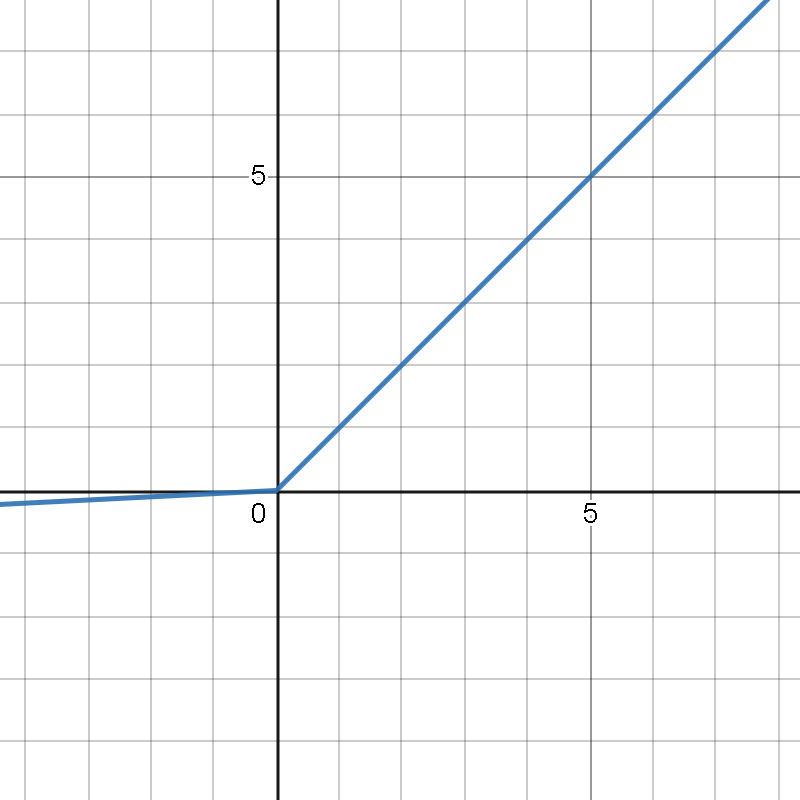Akhil Chaudhary
NIT Warangal graduate, Software Engineer at Wipro.

# Activation FunctionsA neural network would essentially be a weighted linear combination of inputs that can capture linear, simpler patterns in the data but fails miserably while dealing with non-linear, complex patterns. Activation functions help neural networks learn complex representations by introducing non-linearity in the model training.

Activation functions have a major effect on the convergence and thus speed of the neural network: it may speed it up tenfolds or it may never allow it to converge. An activation function also helps to normalize the output of any input in the range between 1 to -1 or 0 to 1.

# TYPES OF ACTIVATION FUNCTIONS:

## SIGMOID

Sigmoid transforms the values between the range 0 and 1 and thus is ideal for binary classifications. The mathematical expression for the sigmoid function is:

f(x) = 1/(1+e^-x)

Implement sigmoid function in Python as below:

import numpy as np

def sigmoid_function(x):

z = (1/(1 + np.exp(-x)))

return z## TANH

The tanh function is quite similar to the sigmoid function. The only difference is that it is symmetric about the origin i.e. the input gets normalized between -1 and +1. The tanh function is:

tanh(x) = 2/(1+e^(-2x)) -1

Implement tanh function in Python as below:

def tanh_function(x):

z = (2/(1 + np.exp(-2*x))) -1

return z## ReLU

ReLU or Rectified Linear Unit can be defined as f(x) = max(0,x). One of its benefits over others is that not all neurons are activated at the same time. Neurons will be deactivated if the output of the linear transformation is less than 0.

Implement ReLU function in Python as below:

def relu_function(x):

if x<0:

return 0

else:

return x## LEAKY ReLU

Leaky ReLU is a special case of ReLU function where the neuron is not deactivated if the output of linear transformation is less than zero. Instead of defining the function as zero for is defined as a very small component of x. It is defined as: f(x) = max(αx, x) where a is a very small quantity.

Implement Leaky ReLU function in Python as below:

def relu_function(x):

if x<0:

return 0.01*x

else:

return x## SOFTMAX

Softmax is a special case of sigmoid function where it is applied to a multi-class classification problem. This function returns the probability for a data point belonging to each individual class.

Implement softmax function in Python as below:

def softmax_function(x):

z = np.exp(x)

z_ = z/z.sum()

return z_

# CONCLUSION

The activation functions described here have their utility and limitations in certain cases. Sigmoid and tanh functions work well for classifiers but suffer from the problem of vanishing gradients: for values much greater than 1 or lesser than -1, the gradient vanishes towards zero, hampering the learning of the network.

ReLU is the most common activation function for hidden layers. If dead neurons affect model performance, Leaky ReLU can be used.

Multi-class outputs can be generated using the softmax function which gives the set of probabilities for the given input to belong to that particular class.